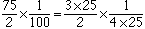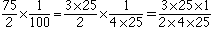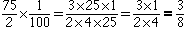75/2 x 1/100 = 3/8 Please show how to came up with the answer? Hi, You need to find a factors that appear in both the numerator and the denominator which you can cancel. With this problem the first fact I see is that 75 = 3 x 25. The second fraction has 100 is the denominator and 100 = 4 x 25. ThusMultiplying fractions involves multiplying the numerators and denominators, henceNow you can cancel the 25's and the product or 75/2 and 1/100 isCheers, Penny Go to Math Central